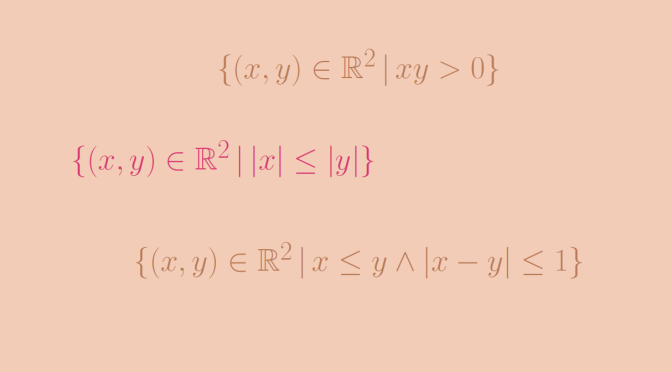# Around binary relations on sets

We are considering here binary relations on a set $$A$$. Let’s recall that a binary relation $$R$$ on $$A$$ is a subset of the cartesian product $$R \subseteq A \times A$$. The statement $$(x,y) \in R$$ is read as $$x$$ is $$R$$-related to $$y$$ and also denoted by $$x R y$$.

Some importants properties of a binary relation $$R$$ are:

reflexive
For all $$x \in A$$ it holds $$x R y$$
irreflexive
For all $$x \in A$$ it holds not $$x R y$$
symmetric
For all $$x,y \in A$$ it holds that if $$x R y$$ then $$y R x$$
antisymmetric
For all $$x,y \in A$$ if $$x R y$$ and $$y R x$$ then $$x=y$$
transitive
For all $$x,y,z \in A$$ it holds that if $$x R y$$ and $$y R z$$ then $$x R z$$

A relation that is reflexive, symmetric and transitive is called an equivalence relation. Let’s see that being reflexive, symmetric and transitive are independent properties.

### Symmetric and transitive but not reflexive

We provide two examples of such relations. For the first one, we take for $$A$$ the set of the real numbers $$\mathbb R$$ and the relation $R = \{(x,y) \in \mathbb R^2 \, | \, xy >0\}.$ $$R$$ is symmetric as the multiplication is also symmetric. $$R$$ is also transitive as if $$xy > 0$$ and $$yz > 0$$ you get $$xy^2 z >0$$. And as $$y^2 > 0$$, we have $$xz > 0$$ which means that $$x R z$$. However, $$R$$ is not reflexive as $$0 R 0$$ doesn’t hold.

For our second example, we take $$A= \mathbb N$$ and $$R=\{(1,1)\}$$. It is easy to verify that $$R$$ is symmetric and transitive. However $$R$$ is not reflexive as $$n R n$$ doesn’t hold for $$n \neq 1$$.

### Reflexive and transitive but not symmetric

We take $$A=\mathbb R$$ and $R = \{(x,y) \in \mathbb R^2 \, | \, \vert x \vert \le \vert y \vert\}.$ One can verify that $$R$$ is reflexive and transitive. It is also not symmetric. It is not antisymmetric either. As a counterexample we have $$\vert -3 \vert \le \vert 3 \vert$$ and $$\vert 3 \vert \le \vert -3 \vert$$. However $$-3 \neq 3$$.

### Reflexive and symmetric but not transitive

Consider $$A=\mathbb R$$ and $R = \{(x,y) \in \mathbb R^2 \, | \, \vert x – y \vert \le 1\}.$ In other word, $$x$$ is $$R$$-related to $$y$$ if and only if the relative distance between $$x$$ and $$y$$ is less than $$1$$ foot. $$R$$ is reflexive and symmetric but not transitive as $$\vert 1 – 0 \vert \le 1$$, $$\vert 2 – 1 \vert \le 1$$ but $$\vert 2 – 0 \vert > 1$$.

A relation that is reflexive, antisymmetric and transitive is called a partial order. Let’s see that being reflexive, antisymmetric and transitive are independent properties.

### Reflexive and transitive but not antisymmetric

We already came across such a binary relation above considering $$A=\mathbb R$$ and $R = \{(x,y) \in \mathbb R^2 \, | \, \vert x \vert \le \vert y \vert\}.$

### Reflexive and antisymmetric but not transitive

Here we take $$A = \mathbb R$$ and $R = \{(x,y) \in \mathbb R^2 \, | \, x \le y \text{ and } \vert x-y \vert \le 1\}.$ $$R$$ is reflexive and antisymmetric as $$\le$$ is a partial order of $$\mathbb R$$. $$R$$ is not transitive as $$0 R 1$$ and $$1 R 2$$ while $$0 R 2$$ doesn’t hold.

### Antisymmetric and transitive but not reflexive

We define on $$\mathbb N$$ the binary relation $$R$$ by: $$a R b$$ if and only if $$a$$ is even and divides $$b$$. If $$a R b$$ and $$b R a$$, $$a$$ and $$b$$ are both even and as the divisibility relation is antisymmetric, $$R$$ is also antisymmetric. For $$a,b,c \in \mathbb N$$, if $$aRb$$ and $$b R c$$ both hold, $$a,b$$ are even and $$a$$ divides $$c$$ by transitivity of the divisibility relation. Hence $$a Rc$$ which proves that $$R$$ is transitive.

However, $$R$$ is not reflexive as $$3 R 3$$ doesn’t hold because $$3$$ is not even.